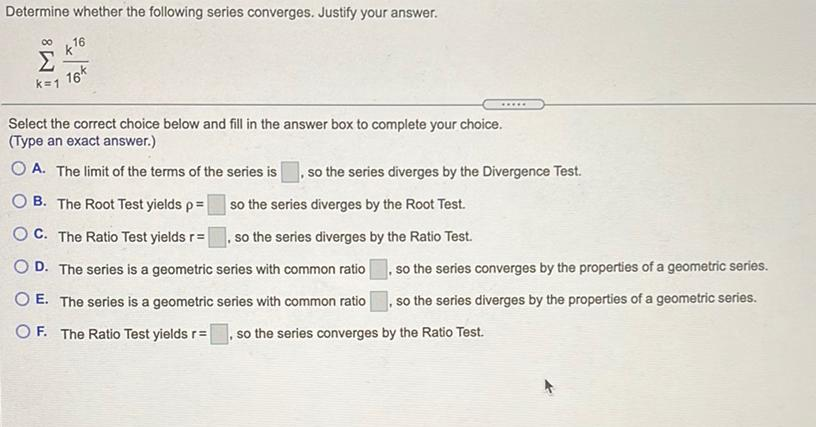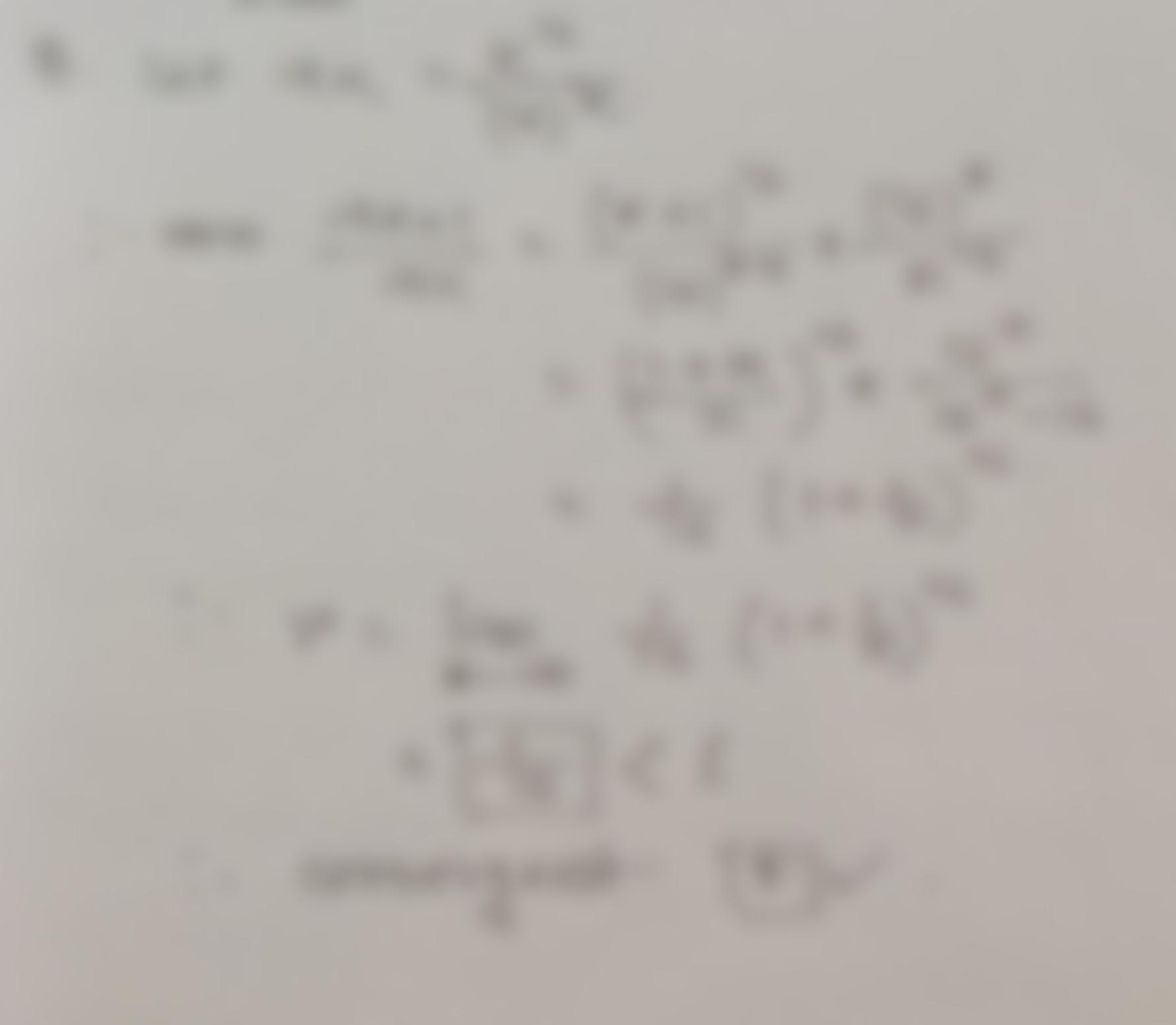Question:

Determine whether the following series converges. Justify your answer. 00 16 k=1 16k Select the correct choice below and fill inDetermine whether the following series converges. Justify your answer. 00 16 k=1 16k Select the correct choice below and fill in the answer box to complete your choice. (Type an exact answer.) O A. The limit of the terms of the series is . so the series diverges by the Divergence Test OB. The Root Test yields pa so the series diverges by the Root Test. C. The Ratio Test yields r= so the series diverges by the Ratio Test. D. The series is a geometric series with common ratio . so the series converges by the properties of a geometric series. O E. The series is a geometric series with common ratio . so the series diverges by the properties of a geometric series. OF. The Ratio Test yields r= , so the series converges by the Ratio Test.